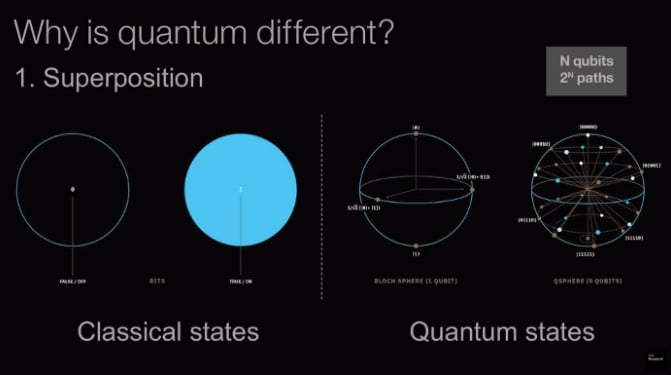News

# Researchers in Japan Claim New Quantum Circuit Can Compute the Fast Fourier Transform

October 20, 2020 by Jake Hertz

## Looking to build upon pre-existing quantum Fourier transform implementations, researchers in Japan have turned to the Fast Fourier Transform.

In classical computing, the Fourier transform is a mathematical operation that is fundamental to fields such as signal processing. In the world of quantum computing, the quantum Fourier transform (QFT) is equally fundamental##### Quantum vs. classical computing. Image used courtesy of Towards Data Science

The QFT is the quantum implementation of the discrete Fourier transform over the amplitudes of a wavefunction. It is an essential part of many quantum algorithms, notably Shor's factoring algorithm and quantum phase estimation. However, researchers feel that there are still improvements that can be made to the QFT to help make it an even more powerful tool.

### Quantum Fast Fourier Transform

The Fourier transform is often implemented by way of the Fast Fourier Transform (FFT) in classical computing. The FFT is a faster computational method of computing the discrete Fourier transform, changing the computational complexity from O(n^2) to O(n logn).

In an attempt to harness the huge advantages of the FFT, researchers in Japan set out to implement an FFT in the quantum domain. Their new quantum FFT (QFFT) is defined as a transformation of the tensor product of quantum states.

This is different from the conventional quantum Fourier transform (QFT), which is defined to be a linear transformation of the amplitudes for the superposition of quantum states.##### A conceptual image of a high pass filter applied to quantum images. Image used courtesy of Asaka et. al

The new QFT consists of several circuits for executing standard arithmetic operations such as a quantum adder, subtractor, and shift operators. Another unique feature of this new circuit is that it leverages the advent of a quantum random access memory (QRAM) to receive significantly better computational complexity than the standard QFT.

### Benefits of the QFFT

One notable advantage of the QFFT is that it does not generate wasted or "garbage qubits," the basic unit of quantum information. With a continually increasing number of qubits in quantum computers, this efficiency is important.

A major advantage of using the QFFT lies in its quantum superposition in which multiple images are processed simultaneously. In cases where the number of images is sufficiently large, the QFFT is considered computationally superior to the QFT.

It is also worth noting that QFFT is highly versatile, applying to all the problems that can be solved by the conventional FFT.

### Why QFFT Matters

With quantum computers around the corner, researchers and engineers are looking for ways to make the transition as seamless as possible.

By creating a versatile algorithm in the QFFT, the researchers in this study aim to simplify the adoption process of quantum algorithms, which can solve the many engineering problems that currently rely on the FFT. Furthermore, as the number of qubits in quantum computing continually increases, computational efficiency will increasingly become a concern. This new circuit is designed to alleviate this concern by eliminating garbage qubits.

If quantum computing really is the future, then work like this may help to ensure that it's going to be a bright one.